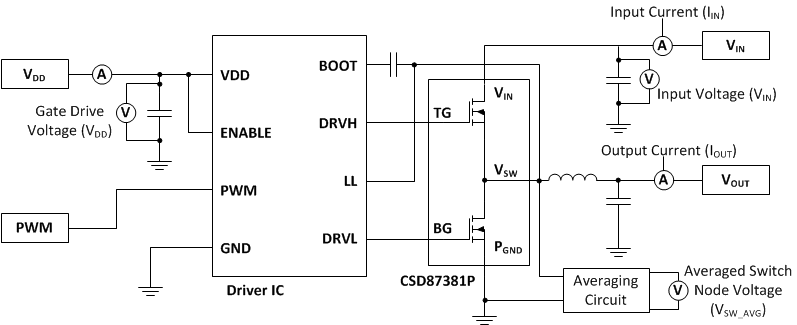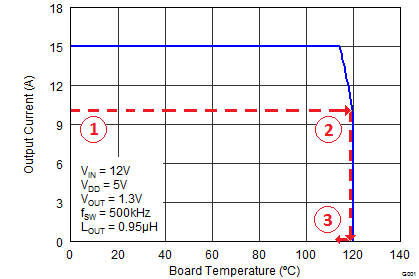SLPS405F March   2013  – March 2015

PRODUCTION DATA.

1. 1Features
2. 2Applications
3. 3Description
4. 4Revision History
5. 5Specifications
6. 6Application and Implementation
1. 6.1 Application Information
7. 7Layout
1. 7.1 Layout Guidelines
2. 7.2 Layout Example
8. 8Device and Documentation Support
9. 9Mechanical, Packaging, and Orderable Information

• MPC|5

## 6 Application and Implementation

NOTE

Information in the following applications sections is not part of the TI component specification, and TI does not warrant its accuracy or completeness. TI ’ s customers are responsible for determining suitability of components for their purposes. Customers should validate and test their design implementation to confirm system functionality.

### 6.1 Application Information

The CSD87381P NexFET power block is an optimized design for synchronous buck applications using 5 V gate drive. The control FET and sync FET silicon are parametrically tuned to yield the lowest power loss and highest system efficiency. As a result, a new rating method is needed, which is tailored towards a more systems-centric environment. System-level performance curves such as Power Loss, Safe Operating Area, and normalized graphs allow engineers to predict the product performance in the actual application.

#### 6.1.1 Power Loss Curves

MOSFET-centric parameters such as RDS(ON) and Qgd are needed to estimate the loss generated by the devices. In an effort to simplify the design process for engineers, TI has provided measured power loss performance curves. Figure 1 plots the power loss of the CSD87381P as a function of load current. This curve is measured by configuring and running the CSD87381P as it would be in the final application (see Figure 27). The measured power loss is the CSD87381P loss and consists of both input conversion loss and gate drive loss. Equation 1 is used to generate the power loss curve.

Equation 1. (VIN × IIN) + (VDD × IDD) – (VSW_AVG × IOUT) = Power Loss

The power loss curve in Figure 1 is measured at the maximum recommended junction temperatures of 125°C under isothermal test conditions.

#### 6.1.2 Safe Operating Curves (SOA)

The SOA curves in the CSD87381P data sheet provides guidance on the temperature boundaries within an operating system by incorporating the thermal resistance and system power loss. Figure 3 to Figure 4 outline the temperature and airflow conditions required for a given load current. The area under the curve dictates the safe operating area. All the curves are based on measurements made on a PCB design with dimensions of 4 inches (W) × 3.5 inches (L) × 0.062 inch (T) and 6 copper layers of 1 oz. copper thickness.

#### 6.1.3 Normalized Curves

The normalized curves in the CSD87381P data sheet provide guidance on the power loss and SOA adjustments based on their application-specific needs. These curves show how the power loss and SOA boundaries adjust for a given set of systems conditions. The primary y-axis is the normalized change in power loss, and the secondary y-axis is the change is system temperature required in order to comply with the SOA curve. The change in power loss is a multiplier for the power loss curve, and the change in temperature is subtracted from the SOA curve.Figure 27. Typical Application

#### 6.1.4 Calculating Power Loss and SOA

The user can estimate product loss and SOA boundaries by arithmetic means (see Design Example). Though the power loss and SOA curves in this data sheet are taken for a specific set of test conditions, the following procedure outlines the steps the user should take to predict product performance for any set of system conditions.

#### 6.1.4.1 Design Example

Operating Conditions:

• Output Current = 8 A
• Input Voltage = 4 V
• Output Voltage = 1 V
• Switching Frequency = 800 kHz
• Inductor = 0.2 µH

#### 6.1.4.2 Calculating Power Loss

• Power Loss at 8 A = 1.44 W (Figure 1)
• Normalized Power Loss for input voltage ≈ 1.06 (Figure 6)
• Normalized Power Loss for output voltage ≈ 0.97 (Figure 7)
• Normalized Power Loss for switching frequency ≈ 1.11 (Figure 5)
• Normalized Power Loss for output inductor ≈ 1.13 (Figure 8)
• Final calculated power loss = 1.44 W × 1.06 × 0.97 × 1.11 × 1.13 ≈ 1.86 W

• SOA adjustment for input voltage ≈ 0.7ºC (Figure 6)
• SOA adjustment for output voltage ≈ –0.3ºC (Figure 7)
• SOA adjustment for switching frequency ≈ 1.03ºC (Figure 5)
• SOA adjustment for output inductor ≈ 1.5ºC (Figure 8)
• Final calculated SOA adjustment = 0.7 + (–0.3) + 1.3 + 1.5 ≈ 2.2ºC

In the previous design example, the estimated power loss of the CSD87381P would increase to 1.86 W. In addition, the maximum allowable board or ambient temperature, or both, would have to decrease by 2.2ºC. Figure 28 graphically shows how the SOA curve would be adjusted accordingly.

1. Start by drawing a horizontal line from the application current to the SOA curve.
2. Draw a vertical line from the SOA curve intercept down to the board or ambient temperature.
3. Adjust the SOA board or ambient temperature by subtracting the temperature adjustment value.

In the design example, the SOA temperature adjustment yields a reduction in allowable board or ambient temperature of 2.2ºC. In the event the adjustment value is a negative number, subtracting the negative number would yield an increase in allowable board or ambient temperature.Figure 28. Power Block SOA